# CLAYFF force field

(Redirected from CLAYFF)

ClayFF is a general force field suitable for the simulation of hydrated and multicomponent mineral systems and their interfaces with aqueous solutions. With the issue of rising atmospheric concentration of the greenhouse (global warming) gas, carbon dioxide (CO2) also comes a burgeoning interest in novel repositories in which to inexpensively "bury" CO2 to reduce its atmospheric load. This issue, among others, has prompted scientists to examine various ubiquitous and inexpensive clays (for example, montmorrillonite or kaolinite) as potential CO2 repositories. But clays are heterogeneous, somewhat unstructured and molecularly complex entities (by comparison to, for example, pure salt --- sodium chloride --- crystals), and there are uncertainties in experimental methods for studying the binding and retention of other atoms, ions, and molecules (such as CO2) to hydrated (water-wettened) clays. Hence, it is important to apply theoretical molecular models to achieve a fundamental atomic-level understanding, interpretation, and prediction of these chemical phenomena. ClayFF is available in molecular simulation codes (for example, MCCCS Towhee and OpenMD) and was developed by Sandia National Laboratories chemist, Randall Cygan, and collaborators at the University of Illinois at Urbana-Champaign. It is suitable for the simulation of hydrated and multicomponent mineral systems and their interfaces with aqueous solutions. The ClayFF approach treats most inter-atomic interactions as being non-bonded. This allows the use of the force field for a wide variety of phases and properly accounts for energy and momentum transfer between the fluid phase and the solid, while keeping the number of parameters small enough to permit modelling of relatively large and highly disordered systems such as clays.

## Functional form

The functional form of ClayFF is given by (Eq. 1 ):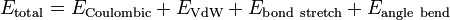$E_{\mathrm {total}} = E_{\mathrm {Coulombic}} + E_{\mathrm {VdW}} + E_{\mathrm {bond~stretch}} + E_{\mathrm {angle~bend}}$

where (Eq. 2 ):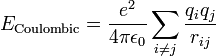$E_{\mathrm {Coulombic}} = \frac{e^2}{4 \pi \epsilon_0} \sum_{i \neq j} \frac{q_i q_j}{r_{ij}}$

(Eq. 3 ):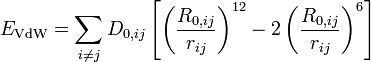$E_{\mathrm {VdW}} = \sum_{i \neq j} D_{0,ij} \left[ \left( \frac{R_{0,ij}}{r_{ij}} \right)^{12} -2 \left( \frac{R_{0,ij}}{r_{ij}}\right)^{6}\right]$

(Eq. 6 ):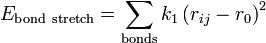$E_{\mathrm {bond~stretch}} = \sum_{\rm {bonds}} k_1\left(r_{ij}-r_0\right)^2$

(Eq. 7 ):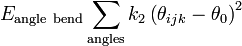$E_{\mathrm {angle~bend}} \sum_{\rm {angles}} k_2 \left(\theta_{ijk} -\theta_{0}\right)^2$